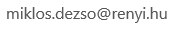﻿ BSM

• Instructor: Dezsö Miklós
• Contact:• Prerequisites: Familiarity with binomial coefficients may be enough in case of exceptionally talented students but at least one course in combinatorics or in graph theory is required in general. A minimum knowledge of linear algebra and discrete probability is advantageous (for Sections 6 and 7).
• Text: The course uses handouts.

Course description: The basic concepts of Combinatorics (counting methods) and Graph and Hypergraph Theory are introduced. Using this framework, classical and relatively new results are discussed from the theory of finite set systems. The emphasis is on studying several successful proof methods including the Linear Algebra and the Probability methods.

Topics to be covered:

• Generating functions (definition, operations on generating functions, applications to counting, binomial theorem, exponential generating functions).
• Recurrences (Fibonacci numbers, derangements, the method of generating functions, Catalan numbers).
• Principle of inclusion and exclusion (the principle and applications, occupancy problems with distinguishable balls and cells, derangements, the number of objects having exactly m properties).
• Symmetric combinatorial structures, block designs (definition, Latin squares, finite projective planes).
• Linear algebra methods - The dimension bound. (Odd town, Fisher inequality, Cross-intersecting hypergraphs.)
• Introductory graph theory (quick overview of fundamental concepts, connectedness, trees; Cayley's theorem on the number of trees).
• Chromatic number and girth (Chromatic number, Graphs from the Hall of Fame - graphs of Zykov, Mycielski, Tutte, Shift graph, Kneser graph.)
• A look at Ramsey theory (Ramsey numbers, Pigeonholes and parties, Existence of Ramsey numbers, Upper bound on R(n), Many colors and non-diagonalramsey numbers, Exact values of Ramsey numbers, A cubic lower bound for R(n),)
• The probability method. (The Erdõs lower bound on R(n), Lower bound for W(k), Tournaments, Large transitive subtournaments, Existence versus construction)
• Proofs by counting. (Antichains, intersecting hypergraphs, 3-chromatic uniform hypergraphs.)